# 6th-8th Grade Algebra: Linear Equations Chapter Exam

Exam Instructions:

Choose your answers to the questions and click 'Next' to see the next set of questions. You can skip questions if you would like and come back to them later with the yellow "Go To First Skipped Question" button. When you have completed the practice exam, a green submit button will appear. Click it to see your results. Good luck!

### Page 1

#### Question 1 1. The equation for the following graph could be _____.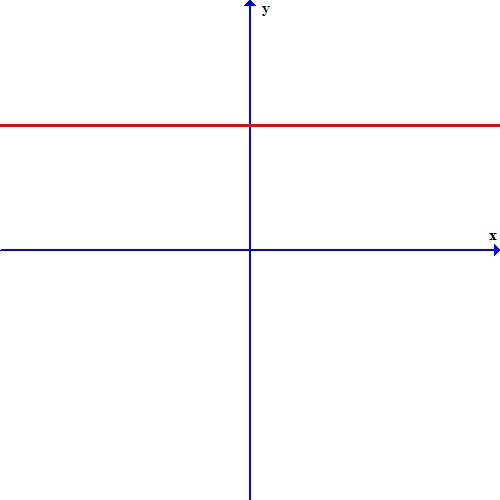#### Question 4 4. If the graph below has the equation Ax + By = -4, what must be true about A and B?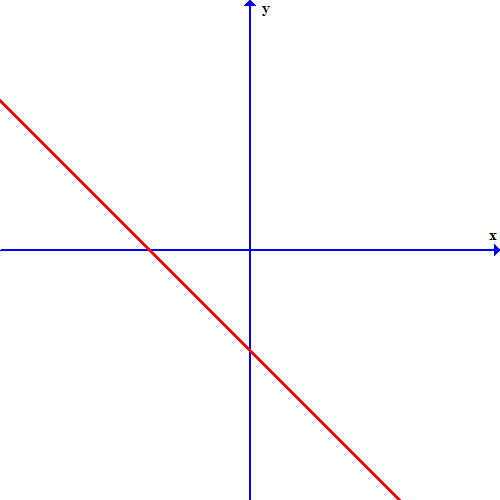### Page 2

#### Question 6 6. What is the slope-intercept form equation of the graph below?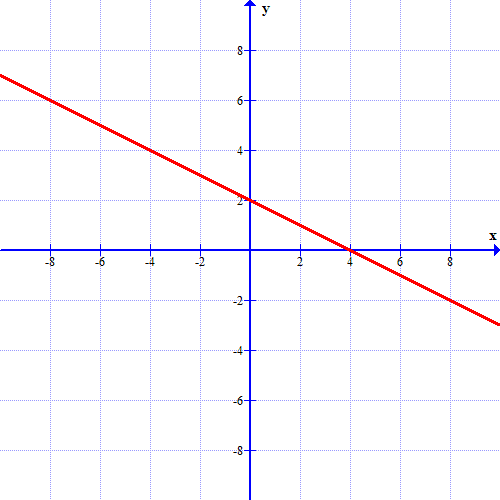### Page 3

#### Question 15 15. What is the equation of the graph below?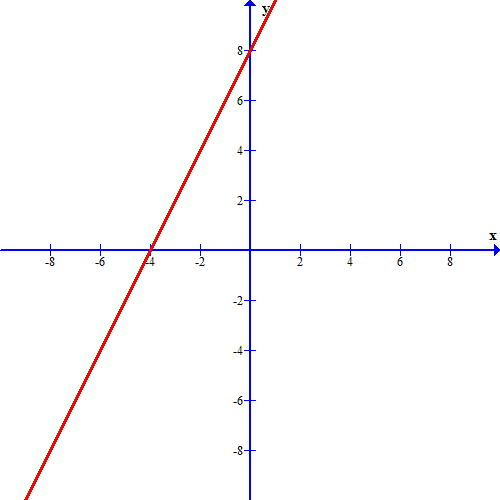### Page 4

#### Question 16 16. If the graph below has the equation Ax+By=C, which statement MUST be true?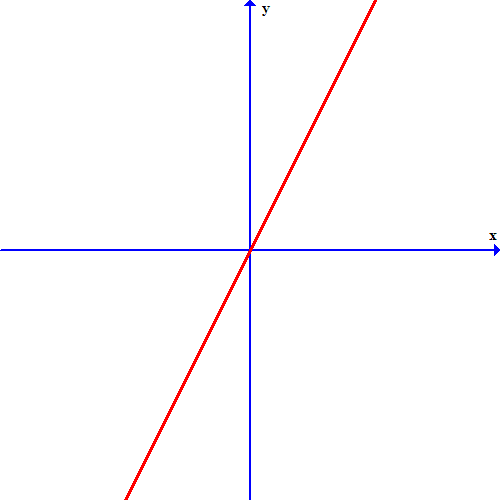#### Question 18 18. If the graph below was written in the slope intercept form, which of the following would be true?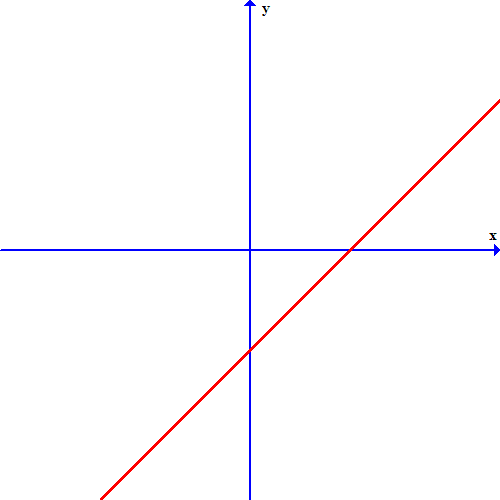### Page 5

#### Question 23 23. Which of these equations represents a line that is perpendicular to the line in the graph below?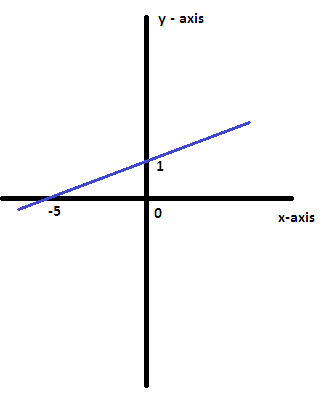#### 6th-8th Grade Algebra: Linear Equations Chapter Exam Instructions

Choose your answers to the questions and click 'Next' to see the next set of questions. You can skip questions if you would like and come back to them later with the yellow "Go To First Skipped Question" button. When you have completed the practice exam, a green submit button will appear. Click it to see your results. Good luck!

Support